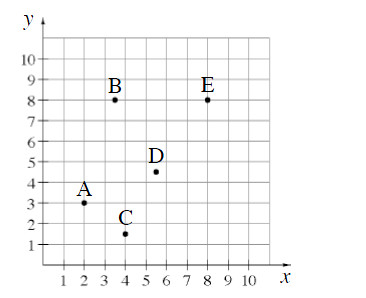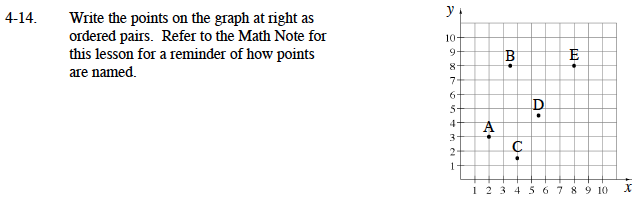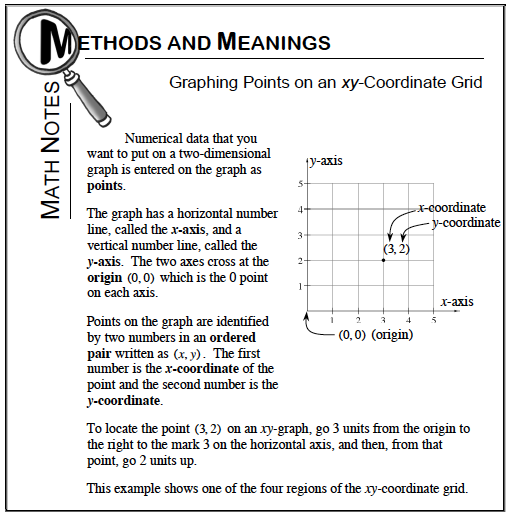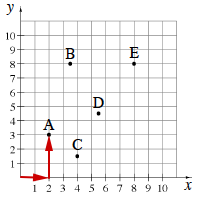### Home > MC1 > Chapter 4 > Lesson 4.1.2 > Problem4-14

4-14.

.Write the points on the graph at right as ordered pairs. Refer to the Math Note for this lesson for a reminder of how points are named. Homework Help ✎From the origin, point A is 2 units to the right and 3 units up. Therefore, point A is at (2,3).Point B is 3.5 units to the right and 8 units up. Therefore, point B is at (3.5, ? ).

Point C is 4 units to the right and 1.5 units up. Therefore, point C is at ( ? , 1.5).

Point D is 5.5 units to the right and 4.5 units up.

Write the ordered pair of point E by using the information given for the other points.